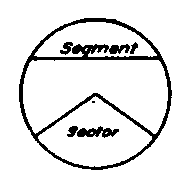A circle is a plane figure bounded by a curved line called the circumference, every point of which is equally distant from a point within called the center, Fig. 58.

A diameter of a circle is a straight line drawn through the center, terminating at both ends in the circumference, Fig. 59.

A radius of a circle is a straight line joining the center with the circumference. All radii of the same circle are equal and their length is always one-half that of the diameter.Fig. 58. Circle.Fig. 59. Diameter and Tangent.An arc is any part of the circumference of a circle. An arc equal to one-half the circumference is called a semi-circumference, and an arc equal to one-quarter of the circumference is called a quadrant, Fig. 60. A quadrant may mean the arc or angle.

A chord, Fig. 61, is a straight line which joins the extremities of an arc but does not pass through the center of the circle.

A secant is a straight line which intersects the circumference in two points, Fig. 61.

A segment of a circle, Fig. 62, is the area included between an arc and a chord.

A sector is the area included between an arc and two radii drawn to the extremities of the arc, Fig. 62.

A tangent is a straight line which touches the circumference at only one point, called the point of tangency or contact, Fig. 59.Fig. 61. Chord and Secant.Fig. 62. Segment and Sector.Fig. 63. Concentric Circles.

Concentric circles are circles having the same center, Fig. 63.

An inscribed angle is an angle whose vertex lies in the circumference and whose sides are chords. It is measured by one-half the intercepted arc, Fig. 64.

A central angle is an angle whose vertex is at the center of the circle and whose sides are radii, Fig. 65.Fig. 64. Inscribed Anglo.Fig. 65. Central Angle.Fig. 66. Inscribed Polygon.

An inscribed polygon is one whose vertices lie in the circumference and whose sides are chords, Fig. 66.